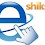# Ncert Cbse Class 8 Mathematics Solutions | Linear Equations in One Variable | NCERT Exercise 2.2 Solved (PART – I)

## CBSE Mathematics Solutions Class VIII

### Ncert Solutions of  8th Maths Exercise 2.2

#### Linear Equations in One Variable

(page 28)
Q 1: If you subtract 1/2 from a number and multiply the result by 1/2, you get 1/8. What is the number?
Solution:
Let the number be x. Then by subtracting 1/2 from x and multiplying the result by 1/2 we get,

Q 2: The perimeter of a rectangular swimming pool is 154 m. Its length is 2m more than twice its breadth. What are the length and breadth of the pool?
Solution:
Let the breadth of the swimming pool be x.
Therefore, its length = 2x + 2
Given the perimeter of the pool = 154 m.
or, 2(2x + 2 + x) = 154
or, 6x + 4 = 154
or,  x = 25m
Length = 2 x 25 + 2 = 52m
Q 3: The base of an isosceles triangle is 4/3 cm. The perimeter of the triangle is 42/15 cm. What is the length of either of the remaining equal sides?
Solution:
Let the length of one equal side of the isosceles triangle be x.
Base of the isosceles triangle = 4/3cm (given)

Q 4: Sum of two numbers is 95. If one exceeds the other by 15, find the numbers.
Solution:
Let one number be x and so, the other number is (x + 15).
or,  x + x + 15 = 95 (sum of the numbers given).
or, x = 40 (and the other number is 40 + 15 = 55)
So, the numbers are 40 and 55 respectively.
Q 5: Two numbers are in the ratio 5 : 3. If they differ by 18, what are the numbers?
Solution:
Let the numbers are x and y
Under the given conditions:

Q 6: Three consecutive integers add up to 51. What are these integers?
Solution:
Let the three consecutive integers be x, (x + 1) and (x + 2).
According to the given condition,
x + (x + 1) + (x + 2) = 51
or, x = 16
Therefore the three consecutive integers are 16, 17 and 18 respectively.
Q 7: The sum of three consecutive multiples of 8 is 888. Find the multiples.
Solution:
Let the first multiple of 8 be 8x.
Second multiple be 8x + 8.
Third multiple be 8x + 8 + 8 = 8x + 16.
According to the given condition,
8x + 8x + 8 + 8x + 16 = 888
or, 24x = 864
or, x = 36.
Hence, 8x = 8 x 36 = 288,
8x + 8 = 296,
8x + 16 = 304.
So, the numbers will be 288, 296 and 304.
Q 8: Three consecutive integers are such that when they are taken in increasing order and multiplied by 2, 3, and 4 respectively, they add up to 74. Find these numbers.
Solution: (Taking hint from Q7 solution do it yourself.)
View solutions of remaining questions visit:

1.2.very healpfull

3.where r answers of rest questions

4.Vaibhav, answers u r looking for will be loaded here soon. Pl be in touch. Tnx 4 writing. Happy surfing and Happy searching !!

5.good it is very useful now if i show the correct sums teacher will say good